# B&C

Founded in 1946, B&C Speakers is a professional loudspeaker transducer manufacturers in Italy.

In addition to designing and distributing components under the B&C brand name, they also supply OEM components to many of the top professional audio brands in the market today.

## no longer sold## B&C DE 75-P

order no. not-available- not available

75 mm driver with high quality cone.

• power handling (continuous/programme) = 80/160 W
• frequency range = 500-18000 Hz
• impedance R = 8 Ohm
• sound pressure level SPL = 108 dB (2,83V; 1m)
• voice coil diameter = 75 mm
• overall diameter d = 170 mm
• mounting depth (not countersunk) t = 67 mm
PDF data sheet## B&C DE 85

order no. not-available- not available

75 mm driver with high quality cone.

• power handling (continuous/programme) = 80/160 W
• frequency range = 500-18000 Hz
• impedance R = 8 Ohm
• sound pressure level SPL = 108 dB (2,83V; 1m)
• voice coil diameter = 75 mm
• overall diameter d = 170 mm
• mounting depth (not countersunk) t = 67 mm
PDF data sheet## B&C DE 700

order no. not-available- not available

75 mm driver with high quality cone.

• power handling (continuous/programme) = 80/160 W
• frequency range = 500-18000 Hz
• impedance R = 8 Ohm
• sound pressure level SPL = 108 dB (2,83V; 1m)
• voice coil diameter = 75 mm
• overall diameter d = 180 mm
• mounting depth (not countersunk) t = 70 mm
PDF data sheet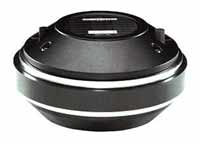## B&C DE 750

order no. not-available- not available

75 mm driver with high quality cone.

• power handling (continuous/programme) = 80/160 W
• frequency range = 500-18000 Hz
• impedance R = 8 Ohm
• sound pressure level SPL = 108,5 dB (2,83V; 1m)
• voice coil diameter = 75 mm
• overall diameter d = 180 mm
• mounting depth (not countersunk) t = 90 mm
PDF data sheet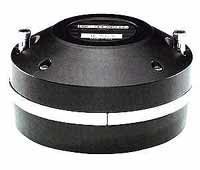## B&C DE 900

order no. not-available- not available

75 mm driver with high quality cone.

• power handling (continuous/programme) = 80/160 W
• frequency range = 500-18000 Hz
• impedance R = 8 Ohm
• sound pressure level SPL = 109,5 dB (2,83V; 1m)
• voice coil diameter = 75 mm
• overall diameter d = 132 mm
• mounting depth (not countersunk) t = 65 mm
PDF data sheet## B&C DE 16

order no. not-available- not available

44 mm driver with high quality cone.

• power handling (continuous/programme) = 30/60 W
• frequency range = 1500-18000 Hz
• impedance R = 8 Ohm
• sound pressure level SPL = 107 dB (2,83V; 1m)
• voice coil diameter = 44 mm
• overall diameter d = 102 mm
• mounting depth (not countersunk) t = 58 mm
PDF data sheet## B&C DE 600

order no. not-available- not available

65 mm driver with high quality cone.

• power handling (continuous/programme) = 80/160 W
• frequency range = 1000-18000 Hz
• impedance R = 8 Ohm
• sound pressure level SPL = 108 dB (2,83V; 1m)
• voice coil diameter = 65 mm
• overall diameter d = 156 mm
• mounting depth (not countersunk) t = 65 mm
PDF data sheet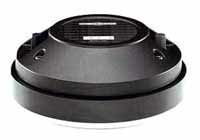## B&C DE 82

order no. not-available- not available

75 mm driver with high quality cone.

• power handling (continuous/programme) = 80/160 W
• frequency range = 500-18000 Hz
• impedance R = 8 Ohm
• sound pressure level SPL = 107 dB (2,83V; 1m)
• voice coil diameter = 75 mm
• overall diameter d = 170 mm
• mounting depth (not countersunk) t = 67 mm
PDF data sheet## B&C ME 15

order no. not-available- not available

Horn for a compression driver with 1 inch throat.

• Throat diameter = 1 inch
• nominal angle (HxV) = 90x60 Deg
• Cut off frequency : 1500 Hz
• over all dimensions (HxWxD) = 138x151x91 mm
• baffle cutout dimensions (HxW) = 109x120 mm
PDF data sheet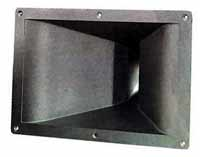## B&C ME 75

order no. not-available- not available

Horn for a compression driver with 2 inch throat.

• Throat diameter = 2 inch
• nominal angle (HxV) = 90x40 Deg
• Cut off frequency : 500 Hz
• over all dimensions (HxWxD) = 268x435x230 mm
• baffle cutout dimensions (HxW) = 222x370 mm
PDF data sheetrecommended cabinet 1:
closed cabinet with 11,3 L volume
from 119/75 Hz (-3dB/-8dB)

recommended cabinet 2:
40 L volume bass reflex cabinet
with HP100 reflex tube, 9 cm long.
from 61/48 Hz (-3dB/-8dB).

## B&C Neodym 10 HPL 51

order no. not-available- not available

25 cm bass driver with high quality cone.

• power handling (continuous/programme) = 150/300 W
• frequency range = 55-4000 Hz
• resonance frequency fs = 55 Hz
• impedance R = 8 Ohm
• sound pressure level SPL = 97,5 dB (2,83V; 1m)
• DC resistance Re = 5 Ohm
• force factor BL = 10,2 N/A
• voice coil inductance L = 1,1 mH
• effective piston radiating area Sd = 320 cm2
• effective mechanical mass incl. air load mms = 24 g
• equivalent volume of compliance Vas = 50 l
• total Q factor Qts = 0,37 (Qms=3,5, Qes=0,41)
• voice coil diameter = 51 mm
• maximum peak linear excursion vibration xlin = +/- 4 mm
• mounting diameter d = 230 mm
• overall diameter d = 261 mm
• mounting depth (not countersunk) t = 124 mm
PDF data sheetrecommended cabinet 1:
closed cabinet with 1,7 L volume
from 260/164 Hz (-3dB/-8dB)

recommended cabinet 2:
2,9 L volume bass reflex cabinet
with HP70 reflex tube, 17 cm long.
from 179/133 Hz (-3dB/-8dB).

## B&C 10 PE 26

order no. not-available- not available

24 cm bass driver with high quality cone.

• power handling (continuous/programme) = 250/500 W
• frequency range = 60-4000 Hz
• resonance frequency fs = 60 Hz
• impedance R = 8 Ohm
• sound pressure level SPL = 99 dB (2,83V; 1m)
• DC resistance Re = 5,7 Ohm
• force factor BL = 17,7 N/A
• voice coil inductance L = 1,25 mH
• effective piston radiating area Sd = 320 cm2
• effective mechanical mass incl. air load mms = 27 g
• equivalent volume of compliance Vas = 37 l
• total Q factor Qts = 0,18 (Qms=6,3, Qes=0,19)
• voice coil diameter = 65 mm
• maximum peak linear excursion vibration xlin = +/- 2,4 mm
• mounting diameter d = 228 mm
• overall diameter d = 260 mm
• mounting depth (not countersunk) t = 110 mm
PDF data sheet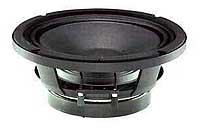recommended cabinet 1:
closed cabinet with 3,5 L volume
from 188/118 Hz (-3dB/-8dB)

recommended cabinet 2:
9,7 L volume bass reflex cabinet
with HP100 reflex tube, 19 cm long.
from 107/81 Hz (-3dB/-8dB).

## B&C 250 AMX

order no. not-available- not available

24 cm bass driver with high quality cone.

• power handling (continuous/programme) = 250/500 W
• frequency range = 65-3500 Hz
• resonance frequency fs = 66 Hz
• impedance R = 8 Ohm
• sound pressure level SPL = 98 dB (2,83V; 1m)
• DC resistance Re = 5,3 Ohm
• force factor BL = 14,2 N/A
• voice coil inductance L = 1,2 mH
• effective piston radiating area Sd = 244 cm2
• effective mechanical mass incl. air load mms = 28 g
• equivalent volume of compliance Vas = 30 l
• total Q factor Qts = 0,28 (Qms=5,3, Qes=0,3)
• voice coil diameter = 65 mm
• maximum peak linear excursion vibration xlin = +/- 2,4 mm
• mounting diameter d = 228 mm
• overall diameter d = 260 mm
• mounting depth (not countersunk) t = 105 mm
PDF data sheetrecommended cabinet 1:
closed cabinet with 16 L volume
from 101/64 Hz (-3dB/-8dB)

recommended cabinet 2:
55 L volume bass reflex cabinet
with 2x HP100 reflex tube, 21 cm long.
from 53/41 Hz (-3dB/-8dB).

## B&C 12 PS 32

order no. not-available- not available

30 cm bass driver with high quality cone.

• power handling (continuous/programme) = 700/1400 W
• frequency range = 45-2000 Hz
• resonance frequency fs = 44 Hz
• impedance R = 8 Ohm
• sound pressure level SPL = 94 dB (2,83V; 1m)
• DC resistance Re = 5,9 Ohm
• force factor BL = 15,9 N/A
• voice coil inductance L = 1,1 mH
• effective piston radiating area Sd = 530 cm2
• effective mechanical mass incl. air load mms = 60 g
• equivalent volume of compliance Vas = 82 l
• total Q factor Qts = 0,35 (Qms=3,2, Qes=0,39)
• voice coil diameter = 100 mm
• maximum peak linear excursion vibration xlin = +/- 6 mm
• mounting diameter d = 282 mm
• overall diameter d = 319 mm
• mounting depth (not countersunk) t = 120 mm
PDF data sheetrecommended cabinet 1:
closed cabinet with 5,3 L volume
from 157/99 Hz (-3dB/-8dB)

recommended cabinet 2:
9,6 L volume bass reflex cabinet
with HP100 reflex tube, 33 cm long.
from 105/78 Hz (-3dB/-8dB).

## B&C 12 PZ 32

order no. not-available- not available

30 cm bass driver with high quality cone.

• power handling (continuous/programme) = 400/800 W
• frequency range = 40-2500 Hz
• resonance frequency fs = 38 Hz
• impedance R = 8 Ohm
• sound pressure level SPL = 95 dB (2,83V; 1m)
• DC resistance Re = 6,2 Ohm
• force factor BL = 21,7 N/A
• voice coil inductance L = 1,1 mH
• effective piston radiating area Sd = 530 cm2
• effective mechanical mass incl. air load mms = 64 g
• equivalent volume of compliance Vas = 102 l
• total Q factor Qts = 0,19 (Qms=5,9, Qes=0,2)
• voice coil diameter = 76 mm
• maximum peak linear excursion vibration xlin = +/- 5,5 mm
• mounting diameter d = 282 mm
• overall diameter d = 316 mm
• mounting depth (not countersunk) t = 134 mm
PDF data sheet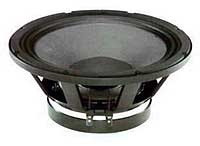recommended cabinet 1:
closed cabinet with 9 L volume
from 155/98 Hz (-3dB/-8dB)

recommended cabinet 2:
22 L volume bass reflex cabinet
with 2x HP100 reflex tube, 23 cm long.
from 93/70 Hz (-3dB/-8dB).

## B&C 12 PL 32

order no. not-available- not available

30 cm bass driver with high quality cone.

• power handling (continuous/programme) = 350/700 W
• frequency range = 50-3500 Hz
• resonance frequency fs = 49 Hz
• impedance R = 8 Ohm
• sound pressure level SPL = 98,5 dB (2,83V; 1m)
• DC resistance Re = 5,3 Ohm
• force factor BL = 16,1 N/A
• voice coil inductance L = 1,1 mH
• effective piston radiating area Sd = 530 cm2
• effective mechanical mass incl. air load mms = 42 g
• equivalent volume of compliance Vas = 97 l
• total Q factor Qts = 0,25 (Qms=6,1, Qes=0,26)
• voice coil diameter = 76 mm
• maximum peak linear excursion vibration xlin = +/- 3,5 mm
• mounting diameter d = 282 mm
• overall diameter d = 316 mm
• mounting depth (not countersunk) t = 134 mm
PDF data sheetrecommended cabinet 1:
closed cabinet with 18 L volume
from 119/75 Hz (-3dB/-8dB)

recommended cabinet 2:
50 L volume bass reflex cabinet
with 2x HP100 reflex tube, 14 cm long.
from 68/52 Hz (-3dB/-8dB).

## B&C 12 PH 32

order no. not-available- not available

30 cm bass driver with high quality cone.

• power handling (continuous/programme) = 250/500 W
• frequency range = 45-4000 Hz
• resonance frequency fs = 42 Hz
• impedance R = 8 Ohm
• sound pressure level SPL = 99,5 dB (2,83V; 1m)
• DC resistance Re = 5,8 Ohm
• force factor BL = 13,3 N/A
• voice coil inductance L = 1,2 mH
• effective piston radiating area Sd = 530 cm2
• effective mechanical mass incl. air load mms = 35 g
• equivalent volume of compliance Vas = 155 l
• total Q factor Qts = 0,28 (Qms=4,3, Qes=0,3)
• voice coil diameter = 65 mm
• maximum peak linear excursion vibration xlin = +/- 3 mm
• mounting diameter d = 282 mm
• overall diameter d = 316 mm
• mounting depth (not countersunk) t = 142 mm
PDF data sheet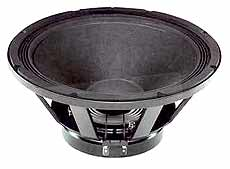recommended cabinet 1:
closed cabinet with 14,9 L volume
from 140/88 Hz (-3dB/-8dB)

recommended cabinet 2:
32 L volume bass reflex cabinet
with 2x HP100 reflex tube, 18 cm long.
from 88/66 Hz (-3dB/-8dB).

## B&C 15 PL 40

order no. not-available- not available

38 cm bass driver with high quality cone.

• power handling (continuous/programme) = 400/800 W
• frequency range = 40-2000 Hz
• resonance frequency fs = 39 Hz
• impedance R = 8 Ohm
• sound pressure level SPL = 99 dB (2,83V; 1m)
• DC resistance Re = 5,1 Ohm
• force factor BL = 20,6 N/A
• voice coil inductance L = 1,2 mH
• effective piston radiating area Sd = 855 cm2
• effective mechanical mass incl. air load mms = 79 g
• equivalent volume of compliance Vas = 211 l
• total Q factor Qts = 0,22 (Qms=8,7, Qes=0,23)
• voice coil diameter = 76 mm
• maximum peak linear excursion vibration xlin = +/- 4 mm
• mounting diameter d = 355 mm
• overall diameter d = 394 mm
• mounting depth (not countersunk) t = 163 mm
PDF data sheetrecommended cabinet 1:
closed cabinet with 21 L volume
from 121/76 Hz (-3dB/-8dB)

recommended cabinet 2:
63 L volume bass reflex cabinet
with 2x HP100 reflex tube, 9 cm long.
from 66/51 Hz (-3dB/-8dB).

## B&C 15 PK 40

order no. not-available- not available

38 cm bass driver with high quality cone.

• power handling (continuous/programme) = 400/800 W
• frequency range = 50-2000 Hz
• resonance frequency fs = 47 Hz
• impedance R = 8 Ohm
• sound pressure level SPL = 99 dB (2,83V; 1m)
• DC resistance Re = 5,1 Ohm
• force factor BL = 19,7 N/A
• voice coil inductance L = 1,1 mH
• effective piston radiating area Sd = 855 cm2
• effective mechanical mass incl. air load mms = 84 g
• equivalent volume of compliance Vas = 140 l
• total Q factor Qts = 0,31 (Qms=5,1, Qes=0,33)
• voice coil diameter = 76 mm
• maximum peak linear excursion vibration xlin = +/- 4 mm
• mounting diameter d = 355 mm
• overall diameter d = 394 mm
• mounting depth (not countersunk) t = 162 mm
PDF data sheetrecommended cabinet 1:
closed cabinet with 28 L volume
from 87/55 Hz (-3dB/-8dB)

recommended cabinet 2:
88 L volume bass reflex cabinet
with 2x HP100 reflex tube, 15 cm long.
from 47/36 Hz (-3dB/-8dB).

## B&C 15 PS 40

order no. not-available- not available

38 cm bass driver with high quality cone.

• power handling (continuous/programme) = 700/1400 W
• frequency range = 35-2000 Hz
• resonance frequency fs = 35 Hz
• impedance R = 8 Ohm
• sound pressure level SPL = 95 dB (2,83V; 1m)
• DC resistance Re = 5,3 Ohm
• force factor BL = 20,4 N/A
• voice coil inductance L = 1,1 mH
• effective piston radiating area Sd = 855 cm2
• effective mechanical mass incl. air load mms = 120 g
• equivalent volume of compliance Vas = 177 l
• total Q factor Qts = 0,32 (Qms=8,5, Qes=0,33)
• voice coil diameter = 100 mm
• maximum peak linear excursion vibration xlin = +/- 6 mm
• mounting diameter d = 355 mm
• overall diameter d = 394 mm
• mounting depth (not countersunk) t = 168 mm
PDF data sheetrecommended cabinet 1:
closed cabinet with 14,3 L volume
from 107/68 Hz (-3dB/-8dB)

recommended cabinet 2:
41 L volume bass reflex cabinet
with 2x HP100 reflex tube, 27 cm long.
from 60/46 Hz (-3dB/-8dB).

## B&C 15 PZB 40

order no. not-available- not available

38 cm bass driver with high quality cone.

• power handling (continuous/programme) = 700/1400 W
• frequency range = 40-2000 Hz
• resonance frequency fs = 39 Hz
• impedance R = 8 Ohm
• sound pressure level SPL = 97 dB (2,83V; 1m)
• DC resistance Re = 5,3 Ohm
• force factor BL = 24,3 N/A
• voice coil inductance L = 1,15 mH
• effective piston radiating area Sd = 855 cm2
• effective mechanical mass incl. air load mms = 123 g
• equivalent volume of compliance Vas = 112 l
• total Q factor Qts = 0,29 (Qms=7,4, Qes=0,3)
• voice coil diameter = 100 mm
• maximum peak linear excursion vibration xlin = +/- 8 mm
• mounting diameter d = 355 mm
• overall diameter d = 394 mm
• mounting depth (not countersunk) t = 171 mm
PDF data sheetrecommended cabinet 1:
closed cabinet with 46 L volume
from 75/47 Hz (-3dB/-8dB)

recommended cabinet 2:
175 L volume bass reflex cabinet
with 2x HP100 reflex tube, 7 cm long.
from 37/30 Hz (-3dB/-8dB).

## B&C Neodym 15 HPL 76W

order no. not-available- not available

38 cm bass driver with high quality cone.

• power handling (continuous/programme) = 350/700 W
• frequency range = 40-2000 Hz
• resonance frequency fs = 38 Hz
• impedance R = 8 Ohm
• sound pressure level SPL = 97 dB (2,83V; 1m)
• DC resistance Re = 6,1 Ohm
• force factor BL = 19,4 N/A
• voice coil inductance L = 1,15 mH
• effective piston radiating area Sd = 855 cm2
• effective mechanical mass incl. air load mms = 114 g
• equivalent volume of compliance Vas = 157 l
• total Q factor Qts = 0,41 (Qms=5,5, Qes=0,44)
• voice coil diameter = 76 mm
• maximum peak linear excursion vibration xlin = +/- 6 mm
• mounting diameter d = 355 mm
• overall diameter d = 394 mm
• mounting depth (not countersunk) t = 171 mm
PDF data sheetrecommended cabinet 1:
closed cabinet with 27 L volume
from 104/65 Hz (-3dB/-8dB)

recommended cabinet 2:
79 L volume bass reflex cabinet
with 2x HP100 reflex tube, 10 cm long.
from 57/44 Hz (-3dB/-8dB).

## B&C Neodym 15 HPL 76

order no. not-available- not available

37 cm bass driver with high quality cone.

• power handling (continuous/programme) = 350/700 W
• frequency range = 40-2000 Hz
• resonance frequency fs = 39 Hz
• impedance R = 8 Ohm
• sound pressure level SPL = 99,5 dB (2,83V; 1m)
• DC resistance Re = 5,4 Ohm
• force factor BL = 19,2 N/A
• voice coil inductance L = 1,7 mH
• effective piston radiating area Sd = 855 cm2
• effective mechanical mass incl. air load mms = 88 g
• equivalent volume of compliance Vas = 194 l
• total Q factor Qts = 0,3 (Qms=4, Qes=0,32)
• voice coil diameter = 76 mm
• maximum peak linear excursion vibration xlin = +/- 4 mm
• mounting diameter d = 354 mm
• overall diameter d = 392 mm
• mounting depth (not countersunk) t = 171 mm
PDF data sheet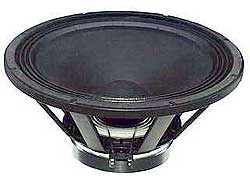recommended cabinet 1:
closed cabinet with 62 L volume
from 70/44 Hz (-3dB/-8dB)

recommended cabinet 2:
192 L volume bass reflex cabinet
with 4x HP100 reflex tube, 28 cm long.
from 38/29 Hz (-3dB/-8dB).

## B&C 18 PS 46

order no. not-available- not available

44 cm bass driver with high quality cone.

• power handling (continuous/programme) = 700/1400 W
• frequency range = 30-2000 Hz
• resonance frequency fs = 28 Hz
• impedance R = 8 Ohm
• sound pressure level SPL = 96,5 dB (2,83V; 1m)
• DC resistance Re = 5,3 Ohm
• force factor BL = 20,8 N/A
• voice coil inductance L = 1,1 mH
• effective piston radiating area Sd = 1134 cm2
• effective mechanical mass incl. air load mms = 153 g
• equivalent volume of compliance Vas = 386 l
• total Q factor Qts = 0,32 (Qms=7,6, Qes=0,33)
• voice coil diameter = 100 mm
• maximum peak linear excursion vibration xlin = +/- 6 mm
• mounting diameter d = 422 mm
• overall diameter d = 460 mm
• mounting depth (not countersunk) t = 191 mm
PDF data sheet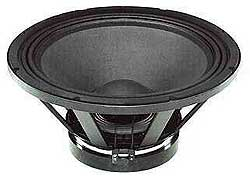recommended cabinet 1:
closed cabinet with 37 L volume
from 78/49 Hz (-3dB/-8dB)

recommended cabinet 2:
135 L volume bass reflex cabinet
with 4x HP100 reflex tube, 33 cm long.
from 40/31 Hz (-3dB/-8dB).

## B&C 18 PZB 46

order no. not-available- not available

44 cm bass driver with high quality cone.

• power handling (continuous/programme) = 700/1400 W
• frequency range = 40-2000 Hz
• resonance frequency fs = 37 Hz
• impedance R = 8 Ohm
• sound pressure level SPL = 97 dB (2,83V; 1m)
• DC resistance Re = 5,3 Ohm
• force factor BL = 24,1 N/A
• voice coil inductance L = 1,15 mH
• effective piston radiating area Sd = 1134 cm2
• effective mechanical mass incl. air load mms = 155 g
• equivalent volume of compliance Vas = 155 l
• total Q factor Qts = 0,37 (Qms=9,4, Qes=0,39)
• voice coil diameter = 100 mm
• maximum peak linear excursion vibration xlin = +/- 8 mm
• mounting diameter d = 422 mm
• overall diameter d = 460 mm
• mounting depth (not countersunk) t = 198 mm
PDF data sheetrecommended cabinet 1:
closed cabinet with 19 L volume

recommended cabinet 2:
63 L volume bass reflex cabinet
with 2x HP100 reflex tube, 8 cm long.
from 64/51 Hz (-3dB/-8dB).

## B&C Coax 12 CXB

order no. not-available- not available

30 cm coax driver with high quality cone.

• power handling (continuous/programme) = 30/60 W
• frequency range = 55-18000 Hz
• resonance frequency fs = 54 Hz
• impedance R = 8 Ohm
• sound pressure level SPL = 104,5 dB (2,83V; 1m)
• DC resistance Re = 5,5 Ohm
• force factor BL = 13,4 N/A
• voice coil inductance L = 1,2 mH
• effective piston radiating area Sd = 522 cm2
• effective mechanical mass incl. air load mms = 35 g
• equivalent volume of compliance Vas = 94,6 l
• total Q factor Qts = 0,35 (Qms=7,2, Qes=0,37)
• voice coil diameter = 44 mm
• maximum peak linear excursion vibration xlin = +/- 2,6 mm
• mounting diameter d = 282 mm
• overall diameter d = 316 mm
• mounting depth (not countersunk) t = 182 mm
PDF data sheet

The cabinet volume calculations are executed considering an external resistance of 0.3 Ohm. We are using, if no other data is known or available, the information supplied by the manufacturer.

Please note: the frequency response doesn't say much about the cut-off frequency inside the cabinet! Therefore, we mostly give you 2 examples with dimensioning considerations and an indication, how low the loudspeaker will reproduce.

Home